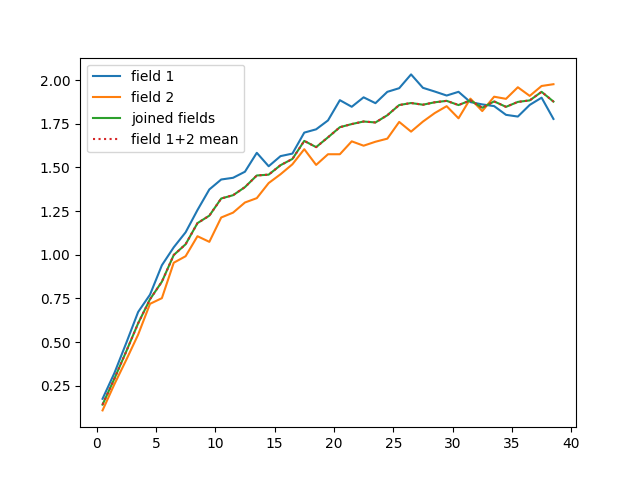Multi-field variogram estimation

In this example, we demonstrate how to estimate a variogram from multiple fields on the same point-set that should have the same statistical properties.

import matplotlib.pyplot as plt
import numpy as np

import gstools as gs

x = np.random.RandomState(19970221).rand(1000) * 100.0
y = np.random.RandomState(20011012).rand(1000) * 100.0
model = gs.Exponential(dim=2, var=2, len_scale=8)
srf = gs.SRF(model, mean=0)

Generate two synthetic fields with an exponential model.

field1 = srf((x, y), seed=19970221)
field2 = srf((x, y), seed=20011012)
fields = [field1, field2]

Now we estimate the variograms for both fields individually and then again simultaneously with only one call.

bins = np.arange(40)
bin_center, gamma1 = gs.vario_estimate((x, y), field1, bins)
bin_center, gamma2 = gs.vario_estimate((x, y), field2, bins)
bin_center, gamma = gs.vario_estimate((x, y), fields, bins)

Now we demonstrate that the mean variogram from both fields coincides with the joined estimated one.

plt.plot(bin_center, gamma1, label="field 1")
plt.plot(bin_center, gamma2, label="field 2")
plt.plot(bin_center, gamma, label="joined fields")
plt.plot(bin_center, 0.5 * (gamma1 + gamma2), ":", label="field 1+2 mean")
plt.legend()
plt.show()Total running time of the script: ( 0 minutes 1.129 seconds)

Gallery generated by Sphinx-Gallery